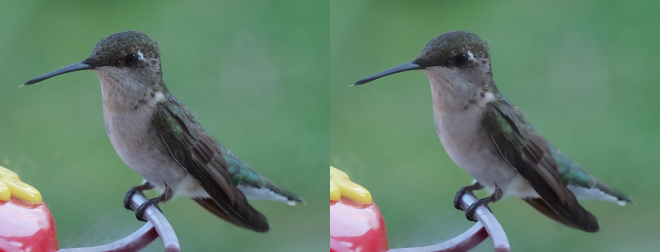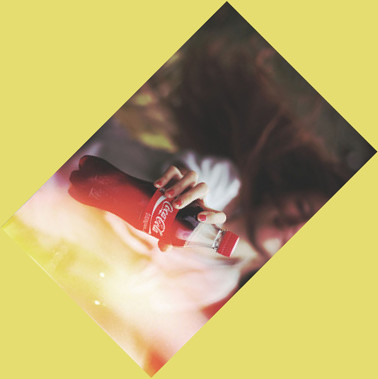# Transformation¶

Note

The image transform.jpg used in this docs is taken by Megan Trace, and licensed under CC BY-NC 2.0. It can be found the original photography from Flickr.

## Enhance¶

New in version 0.5.0.

Reduce the noise of an image by applying an auto-filter. Also see Despeckle.

from wand.image import Image

with Image(filename="hummingbird.jpg") as left:
with left.clone() as right:
right.enhance()
left.extent(width=left.width*2)
left.composite(right, top=0, left=right.width)
left.save(filename="hummingbird-enhance.jpg")## Flip and flop¶

New in version 0.3.0.

You can make a mirror image by reflecting the pixels around the central x- or y-axis. For example, where the given image transform.jpg:The following code flips the image using Image.flip() method:

from wand.image import Image

with Image(filename='transform.jpg') as image:
with image.clone() as flipped:
flipped.flip()
flipped.save(filename='transform-flipped.jpg')


The image transform-flipped.jpg generated by the above code looks like:As like flip(), flop() does the same thing except it doesn’t make a vertical mirror image but horizontal:

from wand.image import Image

with Image(filename='transform.jpg') as image:
with image.clone() as flopped:
flopped.flop()
flopped.save(filename='transform-flopped.jpg')


The image transform-flopped.jpg generated by the above code looks like:## Remap¶

New in version 0.5.3.

Remap replaces all pixels with the closest matching pixel found in the affinity reference image.

from wand.image import Image

with Image(filename="hummingbird.jpg") as left:
with left.clone() as right:
with Image(width=100, height=1, pseudo="plasma:") as affinity:
right.remap(affinity)
left.extent(width=left.width*2)
left.composite(right, top=0, left=right.width)
left.save(filename="hummingbird-remap.jpg")## Rotation¶

New in version 0.1.8.

Image object provides a simple method to rotate images: rotate(). It takes a degree which can be 0 to 359. (Actually you can pass 360, 361, or more but it will be the same to 0, 1, or more respectively.)

For example, where the given image transform.jpg:The below code makes the image rotated 90° to right:

from wand.image import Image

with Image(filename='transform.jpg') as image:
with image.clone() as rotated:
rotated.rotate(90)
rotated.save(filename='transform-rotated-90.jpg')


The generated image transform-rotated-90.jpg looks like:If degree is not multiples of 90, the optional parameter background will help (its default is transparent):

from wand.color import Color
from wand.image import Image

with Image(filename='transform.jpg') as image:
with image.clone() as rotated:
rotated.rotate(135, background=Color('rgb(229,221,112)'))
rotated.save(filename='transform-rotated-135.jpg')


The generated image transform-rotated-135.jpg looks like:## Statistic¶

New in version 0.5.3.

Similar to Spread, but replaces each pixel with the result of a mathematical operation performed against neighboring pixel values.

The type of statistic operation can be any of the following.

• 'gradient'
• 'maximum'
• 'mean'
• 'median'
• 'minimum'
• 'mode'
• 'nonpeak'
• 'root_mean_square'
• 'standard_deviation'

The size neighboring pixels to evaluate can be defined by passing width, and height kwargs.

from wand.image import Image

with Image(filename="hummingbird.jpg") as left:
with left.clone() as right:
right.statistic("median", width=8, height=5)
left.extent(width=left.width*2)
left.composite(right, top=0, left=right.width)
left.save(filename="hummingbird-statistic.jpg")